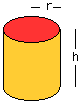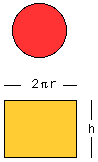Date: Mon, 28 Apr 1997 16:28:21, -0500

Subject: functions

The height of a cylinder is twice the diameter. Express the total surface area as a function of the height h.

Hi Amber

Recently we received a similar question concerning the surface area of a cone. You might find it instructive to look how we answered that question.For a cylinder of height h and radius r you can find the surface area by cutting off the top and bottom then slicing up the side and rolling it flat to get three regions in the plane, two circular disks and a rectangle.Each of the circular disks has area pi r^2 and the rectangle has dimensions 2 pi r by h and hence area 2 pi r h. In your situation you have h = 2 d = 4 r so r = h/4. If you substitute r = h/4 into the expression for the area of each of the three pieces, and add them, you will get the surface area as a function of h.
Cheers,
Harley

Go to Math Central